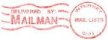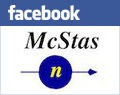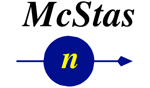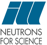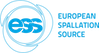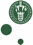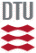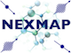McStas: Refractor Component

# The `Refractor` Component

A refractor material/shape, which can be used to model e.g. lenses and prisms.

## Identification

• Author: E. Farhi, B. Cubitt
• Origin: ILL
• Date: Oct 2014
• Version:(Unknown)

## Description

```Single bulk material shape that can be used as a prism or lens.

NEUTRON INTERACTION PROCESSES:
The bulk material can reflect, refract, scatter and absorb neutrons, depending
on the material cross sections and incident angles.

The refracting material is specified from its molar weight, density, coherent
scattering cross section. The refractive index is computed as:
n   = sqrt(1-(lambda*lambda*rho*bc/PI)) is the refraction index

The surface can be coated when specifying a critical wavevector Qc, with e.g.
Qc=m*0.0219 for a super mirror coating. The mirror coating can be suppressed
by setting Qc=0. The critical wavevector is then set to
Qc = 4*sqrt(PI*rho*bc);                                             with
rho= density*6.02214179*1e23*1e-24/weight;
bc = sqrt(fabs(sigma_coh)*100/4/PI)*1e-5;

COMPONENT GEOMETRY:
The component shape can be a sphere, box, cylinder, biconcave spherical lens
or any other shape defined from an external OFF/PLY file.
box:         xwidth, yheight, zdepth
OFF/PLY:     geometry="filename.off or ply", xwidth, yheight, zdepth (bounding box)

The lens_sphere geometry is composed of two concave half spheres of same radius,
separated with a minimal thickness zdepth along the Z axis.

Optionally, you can specify the 'geometry' parameter as a OFF/PLY file name.
The complex geometry option handles any closed non-convex polyhedra.
It computes the intersection points of the neutron ray with the object
transparently, so that it can be used like a regular sample object.
It supports the PLY, OFF and NOFF file format but not COFF (colored faces).
Such files may be generated from XYZ data using:
qhull < coordinates.xyz Qx Qv Tv o > geomview.off
or
powercrust coordinates.xyz
and viewed with geomview or java -jar jroff.jar (see below).

All geometries are centred. The bulk material fills the shape, but can be
set 'outside' when density is given a negative value. In this case, the material
outside the bulk is void (vacuum).

Usually, you should stack more than one of these to get a significant effect
on the neutron beam, so-called 'compound refractive lens'.
The focal length for N lenses with focal 'f' is f/N, where f=R/(1-n)
and R = r/2   for a spherical lens with curvature radius 'r'

COMMON MATERIALS:
Should have high coherent, and low incoherent and absorption cross sections
Be:            density=1.85,  weight=9.0121, sigma_coh=7.63,  sigma_inc=0.0018,sigma_abs=0.0076
Pb:            density=11.115,weight=207.2,  sigma_coh=11.115,sigma_inc=0.003, sigma_abs=0.171
Pb206:                                     sigma_coh=10.68, sigma_inc=0    , sigma_abs=0.03
Pb208:                                     sigma_coh=11.34, sigma_inc=0    , sigma_abs=0.00048
Zr:            density=6.52,  weight=91.224, sigma_coh=6.44,  sigma_inc=0.02,  sigma_abs=0.185
Zr90:                                      sigma_coh=5.1,   sigma_inc=0    , sigma_abs=0.011
Zr94:                                      sigma_coh=8.4,   sigma_inc=0    , sigma_abs=0.05
Bi:            density=9.78,  weight=208.98, sigma_coh=9.148, sigma_inc=0.0084,sigma_abs=0.0338
Mg:            density=1.738, weight=24.3,   sigma_coh=3.631, sigma_inc=0.08,  sigma_abs=0.063
MgF2:          density=3.148, weight=62.3018,sigma_coh=11.74, sigma_inc=0.0816,sigma_abs=0.0822
diamond:       density=3.52,  weight=12.01,  sigma_coh=5.551, sigma_inc=0.001, sigma_abs=0.0035
Quartz/silica: density=2.53,  weight=60.08,  sigma_coh=10.625,sigma_inc=0.0056,sigma_abs=0.1714
Si:            density=2.329, weight=28.0855,sigma_coh=2.1633,sigma_inc=0.004, sigma_abs=0.171
Al:            density=2.7,   weight=26.98,  sigma_coh=1.495, sigma_inc=0.0082,sigma_abs=0.231
Ni:            density=8.908, weight=58.69,  sigma_coh=13.3,  sigma_inc=5.2,   sigma_abs=4.49
Mn: (bc < 0)   density=7.21,  weight=54.94,  sigma_coh=-1.75, sigma_inc=0.4,   sigma_abs=13.3
perfluoropolymer(PTFE/Teflon/CF2):
density=2.2,   weight=50.007, sigma_coh=13.584,sigma_inc=0.0026,sigma_abs=0.0227
Organic molecules with C,O,H,F

Among the most commonly available and machinable materials are MgF2, SiO2, Si, and Al.
```

## Input parameters

Parameters in boldface are required; the others are optional.
Name Unit Description Default
xwidth m width of box 0
yheight m height of box/cylinder 0
zdepth m depth of box 0
geometry str OFF/PLY geometry file name, or NULL to use simple shape A spherical bi-concave lens can be obtained with geometry="lens_sphere" and given radius and zdepth "NULL"
R0 1 Low-angle reflectivity 0.99
sigma_coh barn coherent cross section of refracting material. Use negative value to indicate a negative coherent scattering length 11.74
density g/cm3 density of the refracting material. density < 0 means the material is outside/before the shape. 3.148
weight g/mol molar mass of the refracting material 62.3018
sigma_inc barn incoherent cross section 0
sigma_abs barn thermal absorption cross section 0
Qc Angs-1 critical scattering vector, e.g. Qc=0.0219 for Ni coating. Set Qc=0 to use the bulk critical grazing angles. 0
p_interact 1 MC Probability for scattering the ray; otherwise transmit. Use 0 to compute true probability, or specify it as e.g. 0.05 0.05
RMS Angs root mean square wavyness of the surface 0
focus_scatter deg angle in which to scatter in bulk, with probability 'p_interact' 10
verbose 1 flag to display detailed component behaviour 0
p_scatter 1 flag to allow scattering in the refractor bulk 1
p_reflect 1 flag to allow reflection (grazing angle) at the surface 1
p_refract 1 flag to allow refraction at the refractor surface 1

## Output parameters

Name Unit Description Default
rho
bc
offdata
theta1 deg incoming angle to the surface
theta2 deg outgoing angle to the surface
mean_n
events

• Source code for `Refractor.comp`.
• M. L. Goldberger et al, Phys. Rev. 71, 294 - 310 (1947)
• Sears V.F. Neutron optics. An introduction to the theory of neutron optical phenomena and their applications. Oxford University Press, 1989.
• H. Park et al. Measured operational neutron energies of compound refractive lenses. Nuclear Instruments and Methods B, 251:507-511, 2006.
• J. Feinstein and R. H. Pantell. Characteristics of the thick, compound refractive lens. Applied Optics, 42 No. 4:719-723, 2001.
• Geomview and Object File Format (OFF)
• Java version of Geomview (display only) jroff.jar
• Meshlab can view OFF and PLY files
• qhull for points to OFF conversion
• powercrust for points to OFF conversion

Generated automatically by McDoc, Peter Willendrup <peter.willendrup@risoe.dk> / Tue May 26 13:03:59 2020